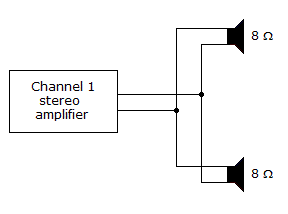# Electronics - Parallel Circuits - Discussion

### Discussion :: Parallel Circuits - General Questions (Q.No.12)

12.In the given circuit, Channel 1 of the stereo amplifier outputs 12 V to the speakers. How much total power is the amplifier delivering to the speakers?

 [A]. 0 W [B]. 18 W [C]. 36 W [D]. More information is needed to find the total power delivered to the speakers.

Explanation:

No answer description available for this question.

 Swathi said: (Dec 5, 2010) p= v*i => v^2/r where r= 8 parellel 8 8*8/(8+8)=4 hence 12*12/4=36

 Navin said: (Dec 22, 2010) p1=24*24/8=18 p2=24*24/8=18 total power=p1+p2=36

 Ilavarasan said: (Jul 18, 2011) p=v*i v=12, i=? R=r1*r2/r1+r2 =8*8/8+8 =64/16 R=4 v=ir i=v/r =12/4 i=3 p=vi p=12*3 p=36

 Ankit V said: (Aug 1, 2011) In given circuit two resistors are in parallel so effective resistance is 4 ohm and we know P=V*V/R. So after calculate P= 36 W

 Dilip said: (Oct 20, 2011) First find current, I=V/R where R=R1*R2/R1+R2 i.e., 8*8/8+8=4ohm I=12/4=3A then, P=VI P=12*3=36 watt.

 Saurabh Kumar said: (Jul 10, 2012) With reference of product over sum. X1X2/X1+X2. R1=8. R2=8. So then, R=R1R2/R1+R2. 8*8/8+8=64/16=4ohm. Given voltage=12v. Then, I=12/4. =3A. So that, P = VI. P = 12*3 = 36W Answer.

 Shaikh said: (Aug 13, 2012) w = v2/R. v =12. Rt = (r1*r2)/(r1+r2) = 4 ohm. w = 144/4. = 36.

 Asif Vellore said: (Apr 12, 2013) Power formula is 3 type: 1) p=i^2*r 2) p=v^2/r 3) p=v*i Take a 2 formula for this question p=V^2/R. FOR 1ST SPEAKER P=144/8 =18W. FOR 2ND SPEAKER P=144/8 =18W. TOTAL POWER OF SPEAKER IS = 36W.

 Roja said: (Jun 10, 2015) p = v*i. v = 12v. i = i1+i2. i1 = v/r1 = 12/8 = 3/2MA. i2 = v/r2 = 12/8 = 3/2MA. i = 3/2+3/2 = 3MA. p = v*i = 12*3 = 36W.

 Harshvardhan Nirmal said: (Jun 12, 2015) p=vi, but v=ir. Therefore. p=i^2r. r=8*8/8+8=4. i=v/r v/r=12/4=3. p=v*(v/r). p=12*3=36W. p=36W.

 Pre said: (Jun 15, 2016) p = i^2 * r. r = r1 * r2/r1 + r2. => 8 * 8/8 + 8, => 64/16, => 4, r = 4. Don't we know I? So that; i = (v/r). p = (v/r)^2 * r, p = ((12/4)^2) * 4, p = (3^2) * 4, p = 9 * 4, p = 36. Then the answer is 36- (c).

 Krunal said: (Nov 4, 2016) p = v * i v = 12, i = ? R = r1 * r2/r1 + r2. = 8 * 8/8 + 8, = 64/16, R = 4. v = ir, i = v/r, = 12/4, i = 3. p = vi. p = 12 * 3, p = 36.